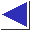# comp.lang.c FAQ list ·Question 3.13

Q: I need to check whether one number lies between two others. Why doesn't

`if(a < b < c)`
work?

A: The relational operators, such as <, are all binary; they compare two operands and return a true or false (1 or 0) result. Therefore, the expression a < b < c compares a to b, and then checks whether the resulting 1 or 0 is less than c. (To see it more clearly, imagine that it had been written as (a < b) < c, because that's how the compiler interprets it.) To check whether one number lies between two others, use code like this:

```	if(a < b && b < c)
```

References: K&R1 Sec. 2.6 p. 38
K&R2 Sec. 2.6 pp. 41-2
ISO Sec. 6.3.8, Sec. 6.3.9
H&S Secs. 7.6.4,7.6.5 pp. 207-210